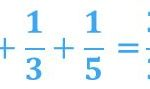Smartick is an advanced online program that teaches kids math and coding in only 15 min. a day

# I want to learn about: Learning Resources

Teaching resources for elementary mathematics from Smartick. Here you will find posts about teaching resources to make learning mathematics easier and more fun.

Feb18

## How to Support Learning Mathematics for 4 Year Olds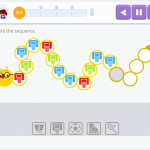Mathematics forms a part of our life practically from the time that we are born. From the time we are very young, without evening realizing, we participate in all types of activities that aid in the development and construction of mathematical thinking. “Humans are born with the fundamental sense of quantity.” – James Geary Presenting […]

Feb11

## Rule of Three for Calculating PercentagesIn earlier posts, we have learned what a percent is and how to calculate it. Today we are going to use the rule of three to solve different types of problems related to percentages. Rule of three to calculate the percentage of a number For example, we want to calculate 30% of 360. 30% means […]

Jan28

## How to Perform Multiplication Problems with an Area Model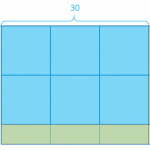In today’s post, we are going to learn how to solve multiplication problems using an area model. You will see how simple it is to understand this multiplication algorithm. Earlier with Smartick, we saw that we can represent multiplication with a rectangle whose sides have multiplication factors. Today I will teach you the area model, based […]

Jan21

## Digital Manipulative Materials Used by the Smartick MethodIt is becoming more and more common to find digital manipulative materials for learning mathematics. At Smartick we are aware that manipulative and pictorial representations are fundamental for the acquisition of some mathematical content. However, these materials should be organized in order to get the most out of them. In other words, the use of […]

Jan17

## The Pomodoro Technique and MathematicsWith our attention spans getting shorter and shorter, there is a lot of advice about how to get rid of distractions. Barbara Oakley, one of the greatest experts in learning how to learn, is a huge fan of the Pomodoro Technique which uses a kitchen timer in the shape of a tomato – from which […]

Jan14

## What Are Solid Geometric Shapes or Bodies?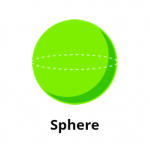Surely, you know about flat geometric figures so today we are going to talk about solid geometric figures which are also known as geometric shapes or bodies. These figures have three dimensions – length, depth, and height. In other words, these figures have volume and we can say that they occupy a place in space. […]

Jan07

## What Do Double and Half Mean?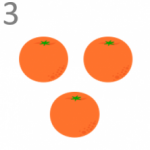In today’s post, we are going to talk about the concepts double and half. First, I will explain what each term means and then we will see a strange situation with them. Double and half explain a type of relationship that exists between two numbers or quantities. Double of a quantity The double of a […]

Dec31

## Divisibility by 4: How Do We Know If a Number Is Divisible by 4?In this post, we are going to learn the criteria for divisibility of the number 4 and understand how they function. The divisibility criteria for the number 4 are rules to know if a number can be divided by 4. They are simple to learn and their explanations are easy to understand. How do we […]

Dec24

## Some Prime Number Curiosities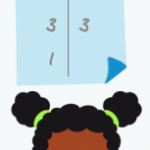For this entry, we are going to learn some surprising facts about prime numbers. We would recommend reading our post about prime numbers and composite numbers first, to refresh your knowledge about these numbers. Are you ready to move on? Perfect! I’m sure that you know it is possible to write some numbers as products of […]

Dec10

## Logic Exercises: Graphs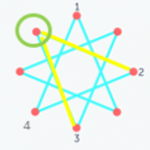In today’s entry, we are going to explain a type of logic exercise that can sometimes get confusing for students. These are logic exercises based on images or graphs and next, we will solve one of the logic exercises that can appear during the Smartick logic sessions. Problem: “The image shows a figure with eight […]

Dec03

## Coordinates: Movements Within the Same Quadrant and Between Them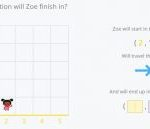We have learned what the coordinates are in this blog post, and about the quadrants in which coordinates are divided. But with the exercises in Smartick, we also work with movement within the same quadrant and between them. The placement of Cartesian coordinates has three elements: The initial position: the coordinate in which it starts. The movement: the […]